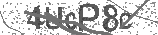﻿ The 3 Times Table Song (Multiplying by 3) | Silly School Songs | Safe Videos for Kids
Welcome

# The 3 Times Table Song (Multiplying by 3) | Silly School Songs

Thanks! Share it with your friends!

URL

You disliked this video. Thanks for the feedback!

Sorry, only registred users can create playlists.URLFind Related Videos  added
60 Views## Description

Kids can learn their 3 times table (multiplying by 3) with this fun song that will get them moving! For more music and information, visit http://sillyschoolsongs.com.

Lyrics:

Let's learn how to multiply by 3
The 3 times table won't you say it with me
Let's learn how to multiply by 3
The 3 times table won't you say it with me
(Let's do it!)

3 x 1 = 3
3 x 2 = 6
3 x 3 = 9
3 x 4 = 12
3 x 5 = 15
3 x 6 = 18
3 x 7 = 21
3 x 8 = 24
3 x 9 = 27
3 x 10 = 30
3 x 11 = 33
3 x 12 = 36

Alright guys! We did it!
We've got one more time.
We're going to turn the numbers around, and do it again.

1 x 3 = 3
2 x 3 = 6
3 x 3 = 9
4 x 3 = 12
5 x 3 = 15
6 x 3 = 18
7 x 3 = 21
8 x 3 = 24
9 x 3 = 27
10 x 3 = 30
11 x 3 = 33
12 x 3 = 36

Great job, everybody!
Now you know how to multiply by 3.
We'll see you next time!Be the first to comment